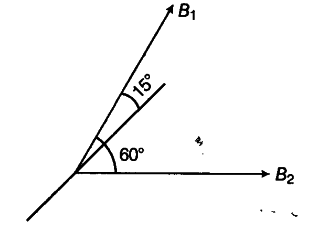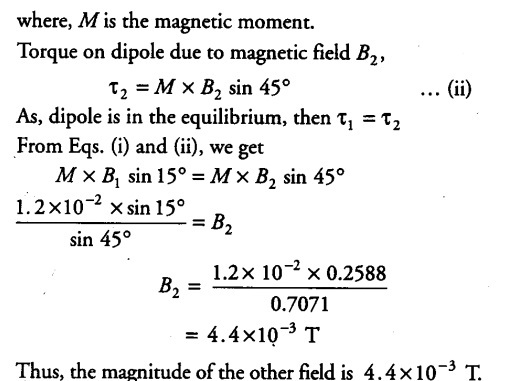# A magnetic dipole is under the influence of two magnetic fields

A magnetic dipole is under the influence of two magnetic fields. The angle between the field directions is 60° and one of the fields has a magnitude of 1.2 x \${{10}^{-2}}\$ T.
If the dipole comes to stable equilibrium at an angle of 15° with this field, what is the magnitude of the other field?

Let one of the magnetic fields be { B }_{ 1 } and other be
{ B }_{ 2 }-
Angle between { B }_{ 1 } and { B }_{ 2 } is 60°.
Given, { B }_{ 1 } =

1.2 x {{10}^{-2}} TDipole is in equilibrium at an angle 15° from { B }_{ 1 } or 60-15 = 45° from

{ B }_{ 2 }

Torque on dipole due to magnetic field { B }_{ 1 },

{ τ}_{ 1 } =

M x { B }_{ 1 } sin15°.